# Xith3D

Xith3D is scenegraph-based Java 3D programming API focused on game creation.

## Xith3D FAQ

Information
none

Operating system used
none

Software prerequisites
none

Procedure
1. Rendering - The process of drawing the geometrical model from its numerical representation, onto the hardware display so that the user can visualize it.

2. Scene Graph - Individual graphics elements in an application are constructed as seperate objects. When these objects are connected together into a treelike structure, this stucture is called a scene graph.

3. Topology - The arrangement in which the vertices of graphics objects (such as a cube) are connected to each other.

4. Xith3D uses the following programming conventions:

• The default coordinate system is right-handed, with +Y (index finger) being up, +X (thumb) horizontal to the right, and +Z (middle) directed toward the viewer.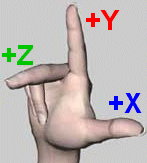• All angles or rotational representations are in radians. A positive rotation is counter clockwise.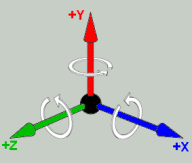• All distances are expressed in units or fractions of meters.

• The face of a polygon is the front face where the vertices are numbered counter clock wise. The front face should be rendered. The opposite side is called the cull face which should not be rendered.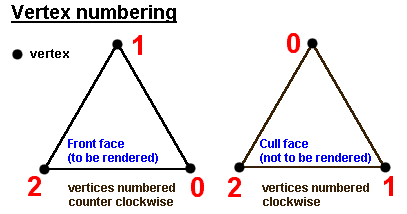5. The TextureLoader is a helper class to create textures out of images. Images can be stored as PNG and JPG. The size (width and height) of an image must be a power of two for rendering efficiency. If this is not the case the image size is scaled to a power of two.

6. To map multiple textures (in the example below 2 textures) to a geometric object, see the following code example:

• Create a geometry object

/**
* Creates a cube.
*/
static public Geometry createCubeViaTriangles(float x, float y, float z, float size)
{
float half = size/2f;

Point3f[] coords = new Point3f[] {

// Triangle A (See image below for explanation)
// vertix a0
new Point3f(-half+x, half+y, half+z),
// vertix a1
new Point3f(half+x, half+y, half+z),
// vertix a2
new Point3f(half+x, half+y, -half+z),

// Triangle B (See image below for explanation)
// vertix b0
new Point3f(half+x, half+y, -half+z),
// vertix b1
new Point3f(-half+x, half+y, -half+z),
// vertix b2
new Point3f(-half+x, half+y, half+z),

// For clarity other code not displayed.

};

TexCoord2f[] texCoords = new TexCoord2f[] {

// assign (0f,0f) to vertix a0
new TexCoord2f(0f,0f),
// assign (1f,0f) to vertix a1
new TexCoord2f(1f,0f),
// assign (1f,1f) to vertix a2
new TexCoord2f(1f,1f),

// assign (1f,1f) to vertix b0
new TexCoord2f(1f,1f),
// assign (0f,1f) to vertix b1
new TexCoord2f(0f,1f),
// assign (0f,0f) to vertix b2
new TexCoord2f(0f,0f),

// For clarity other code not displayed.

};

// - vertexCount (number of vertices) = coords.length
// - vertexFormat = GeometryArray.COORDINATES |
//   GeometryArray.TEXTURE_COORDINATE_2
// - texCoordSetCount (number of texture coordinate sets) = 2
//   in this example the 2 textures are mapped on the geometric object using the
//   same texture coordinates.
// - texCoordSetMap (texture coordinate mapping array) = new int[] {0,1}
//   in this example the first texture coordinate is assigned to array 0
//   the second texture coordinate to array 1.

TriangleArray qa = null;
qa = new TriangleArray(
coords.length,
GeometryArray.COORDINATES | GeometryArray.TEXTURE_COORDINATE_2,
2,
new int[] {0,1});

qa.setCoordinates(0,coords);
qa.setTextureCoordinates(0,0,texCoords);
qa.setTextureCoordinates(1,0,texCoords);

return qa;
}

Texture coordinates are mapped in the s and t space which ranges from 0.0 to 1.0 in both directions. The TexCoord2f class defines the texture coordinates. These are defined as floating point pairs of values that are used to map the corners of the texture image onto the vertices of the face. We then define the indices into this array of values in a similar way to that used for the vertices and normals.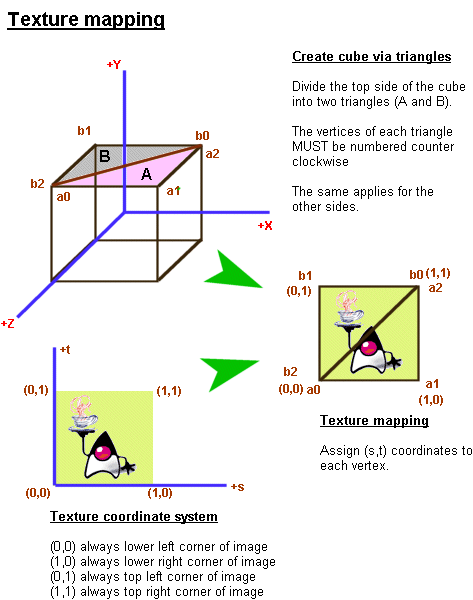7.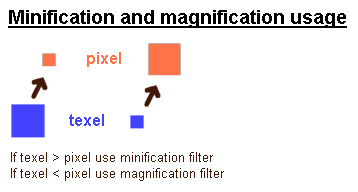8.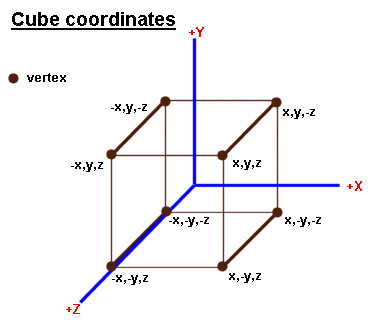9. If you plan to map a texture on a geometry face it is recommended first to determine where the (0,0) texture coordinate is located on your texture image. The second step is to determine where the (0,0) coordinate is mapped on which vertex on the geometry face. This vertex is the starting point (a0). The vertices in the counter clockwise direction are numbered (a0, a1, etc.)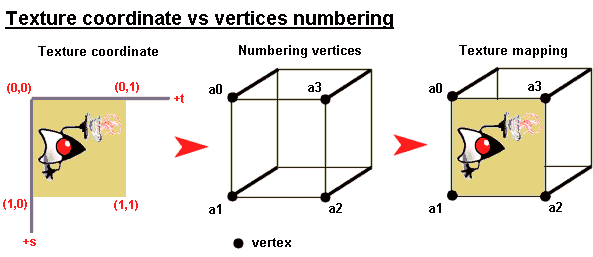TextureLoader.tf.registerPath() should be used when the textures should be searched directly. Each path should end with a "/".

Example: